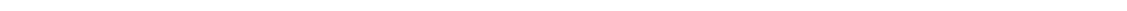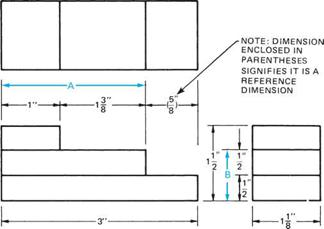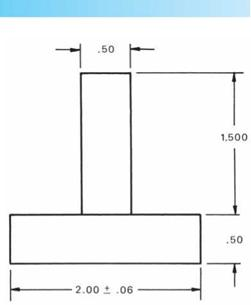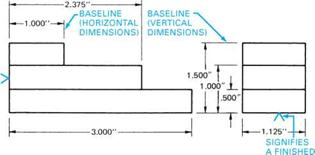## DIMENSIONING IETHODSThere are two basic methods for dimensioning a part: conventional dimensioning and baseline dimensioning.

The conventional method of dimensioning is illustrated by Figure 4.21. In this method, the overall dimen­sions of length, width, and height are given. Dimensions A and B are not given because they are easily found by adding the given dimensions. If dimensions A and/or B were given, they would be identified as reference dimensions. A reference dimension is obtained by adding and/or subtracting other dimensions found on the drawing. A reference dimension is used to expedite reading the drawing; however, it is not a necessary dimen­sion. If a part is dimensioned so that the overall sizes are not given, those dimensions that make up the total must be added to find the overall sizes. Fractional or decimal dimensions may be used, but decimal dimension­ing is preferred.FIGURE 4.21 ■ Conventional dimensioning (A, B are possible reference dimensions).

Baseline dimensioning, Figure 4.22, differs from conventional dimension­ing in that all dimensions originate from baselines. When baseline dimen­sioning is used, the dimensions nor­mally are expressed as three-place decimals.

Baselines are object lines that usu­ally represent machined surfaces, as indicated by the symbol. Baselines

may also originate from centerlines.

The advantage of baseline dimension­ing is that calculations are not required to find a dimension. It is a method com­monly used to dimension precision parts. This method does not give rise to the cumulative error that occurs with conventional dimensioning.FIGURE 4.23 ■ Reference to a basic size dimension.SURFACE FIGURE 4.22 ■ Baseline dimensioning.

Комментарии закрыты.